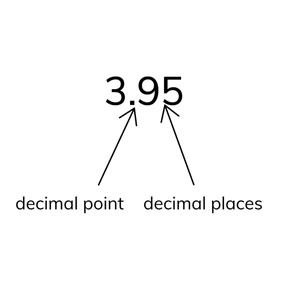Place value- introduction to decimal points

# Place value- introduction to decimal points

Place value- introduction to decimal points8,000 schools use Gynzy92,000 teachers use Gynzy1,600,000 students use Gynzy

## General

Students learn what a decimal number is and what it is used for.

-4.NF.C.5
-4.NF.C.6

## Relevance

Decimal numbers can be seen all around us. Examples are when you buy something for \$2.25 or weigh 61.9 pounds.

## Introduction

Look at the coins and count the total number of cents they represent.

## Development

Explain that decimal numbers are made up of a whole number and a decimal point, followed by more numbers that represent parts of a whole number. The interactive whiteboard shows images of decimal numbers. Discuss with students what the link between decimal numbers and the images are. Explain to students when you can use a decimal number, namely if the object can be split into multiple parts. Tell students that you cannot use a decimal number on whole objects like people or animals.

To check that students understand what decimal numbers are and where they can find them, you can ask the following questions:
- Where can you find decimal numbers?
- Why do you use a decimal number?
- What do the numbers after the decimal point mean?

## Guided practice

Students practice recognizing when to use a decimal number and matching decimal numbers to their situations/images.

## Closing

Play a game in which students must indicate if a number should be a decimal number or not. The interactive whiteboard has images with text that can be read aloud. Students stand if they believe it is a decimal number, and sit down if the number cannot be a decimal number.

## Teaching tips

You can explain the different meanings of numbers in situations if a student finds them overwhelming. Giving the one concrete example of money is a clear base for students who have difficulty understanding decimal numbers, or have trouble finding them around them.

## Instruction materials

You can include objects that use decimal numbers: stopwatch, scale, coins, etc.

### The online teaching platform for interactive whiteboards and displays in schools

• Save time building lessons

• Manage the classroom more efficiently

• Increase student engagement